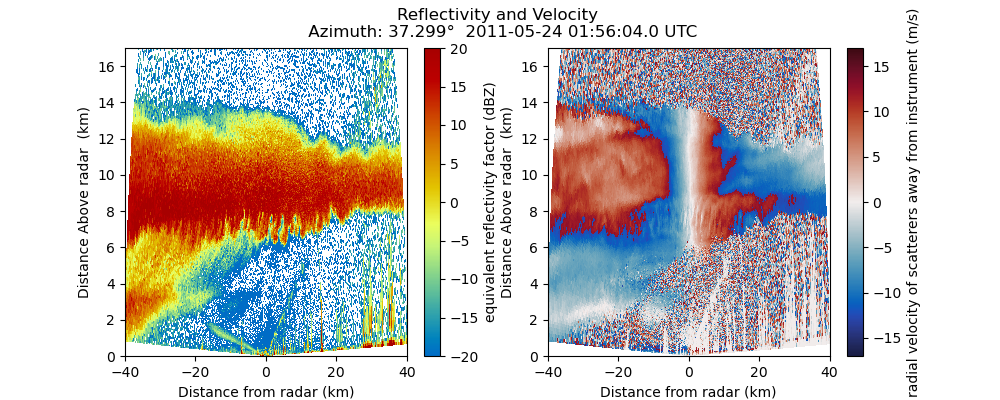# Create a two panel RHI plot#

An example which creates a two panel RHI plot of a cfradial file. The fields included in the two panels are reflectivity and doppler velocity.```print(__doc__)

# Author: Max Grover (mgrover@anl.gov)

import matplotlib.pyplot as plt
import numpy as np

import pyart
from pyart.testing import get_test_data

# Read the data and create the display object
filename = get_test_data("sgpxsaprrhicmacI5.c0.20110524.015604_NC4.nc")

# Fields to plot and ranges
fields_to_plot = ["reflectivity_horizontal", "mean_doppler_velocity"]
ranges = [(-20, 20), (-17.0, 17.0)]
cmaps = ["pyart_HomeyerRainbow", "pyart_balance"]

# Plot the data
nplots = len(fields_to_plot)
plt.figure(figsize=[5 * nplots, 4])

# Plot each field
for plot_num in range(nplots):
field = fields_to_plot[plot_num]
vmin, vmax = ranges[plot_num]
cmap = cmaps[plot_num]

plt.subplot(1, nplots, plot_num + 1)
display.plot(field, 0, vmin=vmin, vmax=vmax, title_flag=False, cmap=cmap)
display.set_limits(ylim=[0, 17])

# Grab the fixed angle and time from the first sweep